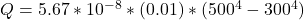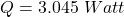## A flat plate of polished copper of surface emissivity 0.1 is 0.1 m long and 0.1 m wide. The plate is placed vertically, with one side heated

Question

A flat plate of polished copper of surface emissivity 0.1 is 0.1 m long and 0.1 m wide. The plate is placed vertically, with one side heated to a surface temperature of 500 K, and the other side remaining insulated. The heated side is exposed to quiescent air at 300 K and the surroundings are also at 300 K. Assume that air can be taken as an ideal gas. Estimate the heat rate from the flat plate.

in progress 0
3 months 2021-09-02T15:26:45+00:00 1 Answers 3 views 0

The heat rate is

Explanation:

From the question we are told that

The surface emissivity is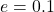The length is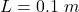The width is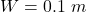The surface temperature of one side is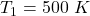The temperature of the quiescent air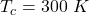The temperature of the surrounding is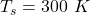The heat rate from the flat plate is mathematically represented as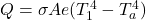Where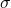is the quiescent air Stefan-Boltzmann constant  and it value is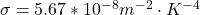A is the area which is mathematically evaluated  as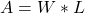substituting values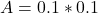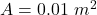substituting values